# Direct sum of connections

## Definition

Suppose$M$ is a differential manifold and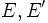$E,E'$ are vector bundles on$M$. Suppose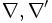$\nabla,\nabla'$ are connections on$E$ and$E'$ respectively. Then, we define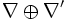$\nabla \oplus \nabla'$ as a connection on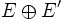$E \oplus E'$ given by: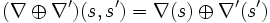$(\nabla \oplus \nabla')(s,s') = \nabla(s) \oplus \nabla'(s')$.

## Facts

### Associativity

Further information: Direct sum of connections is associative upto natural isomorphism

### Commutativity

Further information: Direct sum of connections is commutative upto natural isomorphism

### Distributivity relation with tensor product

Further information: Distributivity relation between direct sum and tensor product of connections

Suppose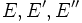$E,E',E''$ are vector bundles over a differential manifold$M$, with connections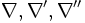$\nabla,\nabla',\nabla''$ respectively. Then, under the natural isomorphism:$E \otimes (E' \oplus E'') \to (E \otimes E') \oplus (E \otimes E'')$

we have an identification between$\nabla \otimes (\nabla' \oplus \nabla'')$ and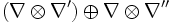$(\nabla \otimes \nabla') \oplus \nabla \otimes \nabla''$. Here,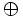$\oplus$ is the direct sum of connections.

An analogous distributivity law identifies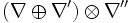$(\nabla \oplus \nabla') \otimes \nabla''$ and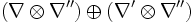$(\nabla \otimes \nabla'') \oplus (\nabla' \otimes \nabla'')$.

### Commutes with dual connection operation

Further information: Direct sum of dual connections equals dual connection to direct sum